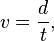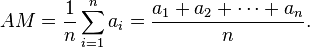Average Speed

# Average Speed

13295
0
SHAREThe indicator’s output is calculated base on mathematical approach.

Calculate Price Speed:

Galileo defined speed as the distance covered per unit of time. (http://en.wikipedia.org/wiki/Speed)

Equation:where v is speed, d is distance and t is time. In this indicator we will define d as distance between price series and t is in minute. We use minute instead of second because second is to fast while sometime we found no price movement for minutes. This could be annoying for some peoples.

Calculate Average Speed of Price:

Indicator calculate Average Speed using Arithmetical  Mean. (http://en.wikipedia.org/wiki/Average, http://en.wikipedia.org/wiki/Arithmetical_mean)

Equation:

If n numbers are given, each number denoted by ai, where i = 1, …, n, the arithmetic mean is the [sum] of the ai’s divided by n orin this indicator n is days, a is speed and AM is output of indicator buffer calculated in point/minute.

Average Speed is a Metatrader 5 (MT5) indicator and the essence of the forex indicator is to transform the accumulated history data.

Average Speed provides for an opportunity to detect various peculiarities and patterns in price dynamics which are invisible to the naked eye.

Based on this information, traders can assume further price movement and adjust their strategy accordingly.

How to install Average Speed.mq5?

• Copy Average Speed.mq5 to your Metatrader Directory / experts / indicators /
• Select Chart and Timeframe where you want to test your indicator
• Right click on Average Speed.mq5
• Attach to a chart
• Modify settings or press ok
• Indicator Average Speed.mq5 is available on your Chart

• Select the Chart where is the Indicator running in your Metatrader Client
• Right click into the Chart
• “Indicators list”
• Select the Indicator and delete